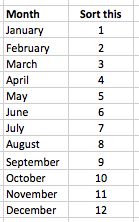# Convert a Month name into a number

This lesson shows you a formula to convert a month name into its corresponding number (i.e. Jan = 1, Feb =2, etc).

There are two common scenarios where this can be useful:

• You have a column of month values entered as text, and you want to sort the list by date order rather than by alphabetically by month name:• You have imported date values from another system. These dates have been imported as text, and you need to convert them from text to dates. The imported dates include the month name rather than the month number, and the MONTH function doesn't know how to convert text to a number, such as Sep and April in the following examples:
• Sep 11 2015 1:50PM
• April 1, 2010 3:02:43 PM EDT

This simple formula will convert these text values into their corresponding numbers:

=MONTH(A1&" 1") (assuming our date has been entered into A1)

Here's how this formula works:

• The MONTH function tells you the month number for a given date. It is commonly used to calculate which month a date represents, e.g.
• April 19, 2014 is stored by Excel as 42113
• =MONTH(42113) returns 4
• The number, 42113 is how Excel stores April 19, 2014 as a date.
• You could also write =MONTH(A1) where A1 contains the date you want to convert.
• In our example, the date has been provided as text. Excel doesn't recognise it as a valid date, so the MONTH doesn't work.
• However, if we concatenate our date text with " 1", we can fool Excel into thinking it's looking at a valid date, so the MONTH function returns the correct value:
• =MONTH("Sep 1") > returns 9
• =MONTH("April 1") > returns 4

Let's return to our challenge of sorting our months by date order rather than alphabetically. To solve this problem you can:

• Sort your data based on that column rather than on the Date column.If you have a more complex scenario, such as the date examples shown above, check out our lesson on converting text values to dates.